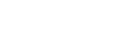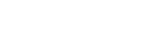# What is Meant by Differentiation in Mathematics?

Differentiation comes down to figuring out how one variable changes with respect to another variable. If this change is a constant (as we have in a line), this concept becomes very similar to the idea of a slope.  But calculus is all about curves, and differentiation allows us to figure out rates of change when this change is itself changing.

## An Example of Differentiation

The best way to look at differentiation is to look at a real-world example.  Let us take the old physics question that asks us to model a car starting at 30 meters per second, but slamming on the breaks.  Intuitively, we should know something about the velocity and acceleration.  Calculus and the derivative will allow us to model this mathematically, and figure out what’s changing at any point.

If you’ve taken a physics class, you should be able to understand the following equation:where x(t) is our function for a position at any time t, in seconds.  Our initial speed is 30 meters per second, and every second we’re slowing down by 5 meters per second.  I’ve graphed the function below:Clearly, our position is not a linear function (it is quadratic).  On our x-axis, we have time (in seconds), and our y-axis, we have position.  How fast our position changes with respect to time (distance/time) is a rate of change more commonly referred to as velocity (or speed).  Now, if this was a linear function, our velocity would simply be the slope of the graph.  However, since it is quadratic, we need to take the derivative.As we can see, our velocity is slowly decreasing.  At t = 0, our velocity is 30 meters per second, but eventually goes to 0 by the 3rd second.

If we want to look at our rate of change of our rate of change, what we will see is how our velocity changes with respect to time as well.  Our change in velocity per second is more commonly referred to as acceleration.  Since our velocity time graph is linear, our derivative will be the same as our slope in this case.Our acceleration is -10 m/s2.  Our we can say that we’re slowing down 10 m/s every second.

The derivative is an important tool in determining how variables change with respect to each other.  AP Calculus AB primarily deals with two dimensions, but we can also use derivatives to compare multiple dimensions.

#### Improve your SAT or ACT score, guaranteed. Start your 1 Week Free Trial of Magoosh SAT Prep or your 1 Week Free Trial of Magoosh ACT Prep today!## Author

•Zachary is a former mechanical engineer and current high school physics, math, and computer science teacher. He graduated from McGill University in 2011 and spent time in the automotive industry in Detroit before moving into education. He has been teaching and tutoring for the past five years, but you can also find him adventuring, reading, rock climbing, and traveling whenever the opportunity arises.